## 5.4.3 Randomized Potential Fields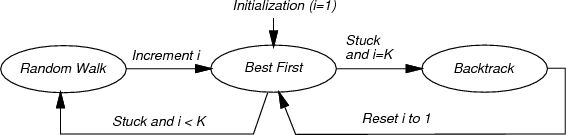Adapting the discrete algorithms from Section 2.2 works well if the problem can be solved with a small number of points. The number of points per axis must be small or the dimension must be low, to ensure that the number of points,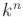, forpoints per axis anddimensions is small enough so that every vertex in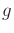can be reached in a reasonable amount of time. If, for example, the problem requires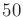points per axis and the dimension is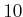, then it is impossible to search all of the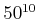samples. Planners that exploit best-first heuristics might find the answer without searching most of them; however, for a simple problem such as that shown in Figure 5.13a, the planner will take too long exploring the vertices in the bowl.5.12

The randomized potential field [70,72,588] approach uses random walks to attempt to escape local minima when best-first search becomes stuck. It was one of the first sampling-based planners that developed specialized techniques beyond classical discrete search, in an attempt to better solve challenging motion planning problems. In many cases, remarkable results were obtained. In its time, the approach was able to solve problems up to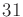degrees of freedom, which was well beyond what had been previously possible. The main drawback, however, was that the method involved many heuristic parameters that had to be adjusted for each problem. This frustration eventually led to the development of better approaches, which are covered in Sections 5.4.4, 5.5, and 5.6. Nevertheless, it is worthwhile to study the clever heuristics involved in this earlier method because they illustrate many interesting issues, and the method was very influential in the development of other sampling-based planning algorithms.5.13

The most complicated part of the algorithm is the definition of a potential function, which can be considered as a pseudometric that tries to estimate the distance from any configuration to the goal. In most formulations, there is an attractive term, which is a metric onthat yields the distance to the goal, and a repulsive term, which penalizes configurations that come too close to obstacles. The construction of potential functions involves many heuristics and is covered in great detail in . One of the most effective methods involves constructing cost-to-go functions over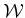and lifting them to. In this section, it will be sufficient to assume that some potential function,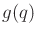, is defined, which is the same notation (and notion) as a cost-to-go function in Section 2.2.2. In this case, however, there is no requirement thatis optimal or even an underestimate of the true cost to go.

When the search becomes stuck and a random walk is needed, it is executed for some number of iterations. Using the discretization procedures of Section 5.4.2, a high-resolution grid (e.g.,points per axis) is initially defined. In each iteration, the current configuration is modified as follows. Each coordinate,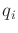, is increased or decreased by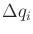(the grid step size) based on the outcome of a fair coin toss. Topological identifications must be respected, of course. After each iteration, the new configuration is checked for collision, or whether it exceeds the boundary of(if it has a boundary). If so, then it is discarded, and another attempt is made from the previous configuration. The failures can repeat indefinitely until a new configuration inis obtained.

The resulting planner can be described in terms of a three-state machine, which is shown in Figure 5.15. Each state is called a mode to avoid confusion with earlier state-space concepts. The VSM and LPM are defined in terms of the mode. Initially, the planner is in the BEST FIRST mode and uses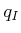to start a gradient descent. While in the BEST FIRST mode, the VSM selects the newest vertex,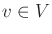. In the first iteration, this is. The LPM creates a new vertex,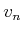, in a neighborhood of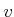, in a direction that minimizes. The direction sampling may be performed using randomly selected or deterministic samples. Using random samples, the sphere sampling method from Section 5.2.2 can be applied. After some number of tries (another parameter), if the LPM is unsuccessful at reducing, then the mode is changed to RANDOM WALK because the best-first search is stuck in a local minimum of.

In the RANDOM WALK mode, a random walk is executed from the newest vertex. The random walk terminates if eitheris lowered or a specified limit of iterations is reached. The limit is actually sampled from a predetermined random variable (which contains parameters that also must be selected). When the RANDOM WALK mode terminates, the mode is changed back to BEST FIRST. A counter is incremented to keep track of the number of times that the random walk was attempted. A parameter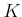determines the maximum number of attempted random walks (a reasonable value is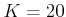). If BEST FIRST fails afterrandom walks have been attempted, then the BACKTRACK mode is entered. The BACKTRACK mode selects a vertex at random from among the vertices in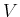that were obtained during a random walk. Following this, the counter is reset, and the mode is changed back to BEST FIRST.

Due to the random walks, the resulting paths are often too complicated to be useful in applications. Fortunately, it is straightforward to transform a computed path into a simpler one that is still collision-free. A common approach is to iteratively pick pairs of points at random along the domain of the path and attempt to replace the path segment with a straight-line path (in general, the shortest path in). For example, suppose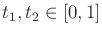are chosen at random, and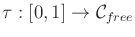is the computed solution path. This path is transformed into a new path,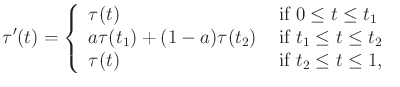(5.39)

in which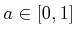represents the fraction of the way from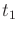to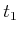. Explicitly,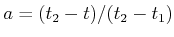. The new path must be checked for collision. If it passes, then it replaces the old path; otherwise, it is discarded and a new pair,, is chosen.

The randomized potential field approach can escape high-dimensional local minima, which allow interesting solutions to be found for many challenging high-dimensional problems. Unfortunately, the heavy amount of parameter tuning caused most people to abandon the method in recent times, in favor of newer methods.

Steven M LaValle 2020-08-14